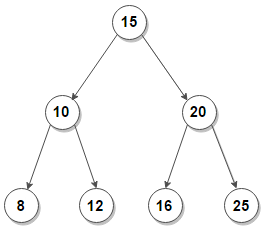# Find K’th smallest and K’th largest element in BST

Given a Binary Search Tree and a positive number K, find K’th smallest and K’th largest element in BST.

For example, consider below binary search tree. If k = 2, the k’th smallest element is 10 and k’th largest element is 20.#### Finding K’th Smallest element –

We know that an in-order traversal of a binary search tree returns the nodes in ascending order. Thus, to find k’th smallest element, we can perform in-order traversal and store the inorder order in an array. Finally we traverse the array and return k’th element from starting. The problem with this approach is that it takes extra space.

We can solve this problem without using any extra space by keeping track of number of nodes processed so far while traversing the tree in in-order fashion. When the number of nodes becomes equal to K, the current node is k’th smallest.

Below is C++/Java implementation of the idea:

Output:

10

## Java

Output:

10

The time complexity of above solution is O(n)

#### Finding K’th Largest element –

We can find k’th largest element using above solution. The idea is to calculate number of nodes n present in the BST. Then kth largest element would be the (n-k)th smallest element.

Above approach requires two traversals of the array. We can solve this problem in one traversal of the array by using reverse in-order traversal (traverse right subtree before left subtree for every node). Then the reverse in-order traversal of a binary search tree will process the nodes in descending order.

Below is C++/Java implementation of the idea:

Output:

20

## Java

Output:

20

The time complexity of above solution is O(n).(1 votes, average: 5.00 out of 5)Loading...